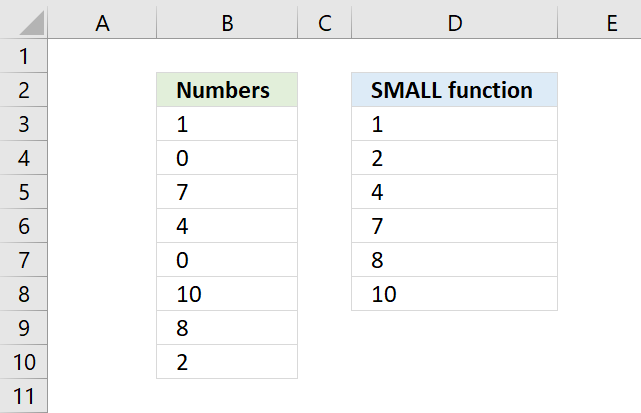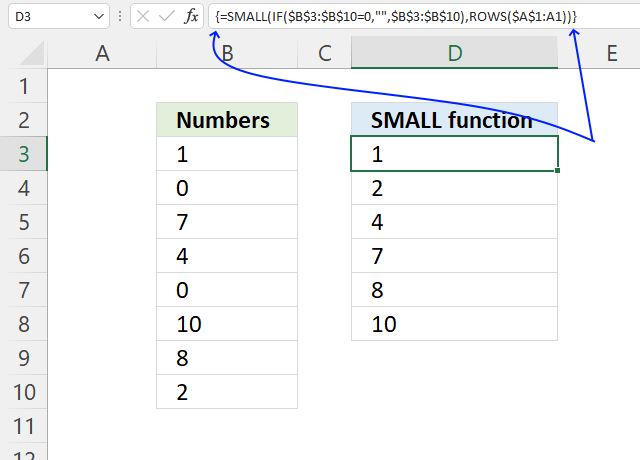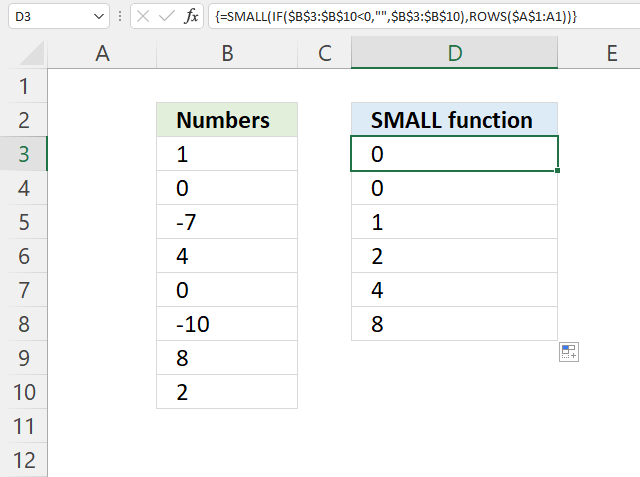Author: Oscar Cronquist Article last updated on December 28, 2021This article shows how to create a formula that sorts numbers from small to large excluding zeros. I will also demonstrate a formula ignoring negative values and then sort from small to large.

## 1. How to ignore zeros using the SMALL function (array formula)The formula in cell D3 is an array formula, it will extract the k-th smallest value ignoring zeros.

=SMALL(IF(\$B\$3:\$B\$10=0, "", \$B\$3:\$B\$10), ROWS(\$A\$1:A1))

### 1.1 How to enter an array formulaExcel 365 users can ignore this, simply enter the formula as a regular formula or even better, use the formula in section 3 below.

To enter an array formula, type the formula in a cell then press and hold CTRL + SHIFT simultaneously, now press Enter once. Release all keys.

The formula bar now shows the formula with a beginning and ending curly bracket telling you that you entered the formula successfully. Don't enter the curly brackets yourself.

### 1.2 Explaining formula

#### Step 1 - Logical test

The equal sign lets you compare value to value, the result is a boolean value TRUE or FALSE. This is called a logical expression or a logical test. We will use this in the next step to filter out numbers that don't meet the condition.

\$B\$3:\$B\$10=0

becomes

{1; 0; 7; 4; 0; 10; 8; 2}=0

and returns

{FALSE; TRUE; FALSE; FALSE; TRUE; FALSE; FALSE; FALSE}.

#### Step 2 - Replace TRUE with the corresponding value

The IF function then returns the corresponding values from column D if TRUE and a blank "" if FALSE.

IF(logical_test, [value_if_true], [value_if_false])

IF(\$B\$3:\$B\$10=0, "", \$B\$3:\$B\$10)

becomes

IF({FALSE; TRUE; FALSE; FALSE; TRUE; FALSE; FALSE; FALSE},"",{1; 0; 7; 4; 0; 10; 8; 2})

and returns

.{1; ""; 7; 4; ""; 10; 8; 2}

If the value is 0 (zero) the IF function returns a blank "" in that position in the array and if not 0 (zero) the IF function returns the number.

#### Step 3 - Replace TRUE with the corresponding value

The SMALL function returns the k-tk smallest value the array determined by ROWS(\$A\$1:A1).

SMALL(array, k)

SMALL(IF(\$B\$3:\$B\$10=0, "", \$B\$3:\$B\$10), ROWS(\$A\$1:A1))

becomes

SMALL({1; ""; 7; 4; ""; 10; 8; 2}, ROWS(\$A\$1:A1))

The ROWS function makes this formula dynamic, in cell D3 the ROWS function returns 1. Copy the cell to next cell below and it changes to ROWS(\$A\$1:A2) and returns 2 making it return the second smallest value in the array in cell D4.

SMALL({1; ""; 7; 4; ""; 10; 8; 2}, ROWS(\$A\$1:A1))

becomes

SMALL({1; ""; 7; 4; ""; 10; 8; 2}, 1)

and returns 1 in cell D3.

## 2. How to ignore zeros using the SMALL function (regular formula)If you want to avoid an array formula then try this formula in cell D3:

=AGGREGATE(15, 6, \$B\$3:\$B\$10/(\$B\$3:\$B\$10<>0), ROWS(\$A\$1:A1))

### 2.1 Explaining formula

#### Step 1 - Find numbers not equal to 0 (zero)

The less than and the larger than signs combined lets you test if a value is not equal to a condition, in this case, 0 (zero).

\$B\$3:\$B\$10<>0

becomes

{1; 0; 7; 4; 0; 10; 8; 2}<>0

and returns

{TRUE; FALSE; TRUE; TRUE; FALSE; TRUE; TRUE; TRUE}.

#### Step 2 - Divide numbers by a boolean array

The forward slash character lets you divide a number by another number in an Excel formula. This works fine with boolean values TRUE and FALSE as well.

TRUE = 1
FALSE = 0 (zero)

\$B\$3:\$B\$10/(\$B\$3:\$B\$10<>0)

becomes

\$B\$3:\$B\$10/{TRUE; FALSE; TRUE; TRUE; FALSE; TRUE; TRUE; TRUE}

becomes

{1; 0; 7; 4; 0; 10; 8; 2}/{TRUE; FALSE; TRUE; TRUE; FALSE; TRUE; TRUE; TRUE}

and returns

{1; #DIV/0!; 7; 4; #DIV/0!; 10; 8; 2}.

Notice how some values are actually error values in the array above. This is because when you divide with FALSE which is equivalent with 0 (zero) and that returns a #DIV/0! error. It is not possible to divide a number by zero.

#### Step 3 - Extract k-th smallest number

The AGGREGATE function performs different specific functions to a list or database.

Array form

AGGREGATE(function_numoptionsarray, [k])

function_num - 15 (SMALL function)

options - 6 (ignore error values)

AGGREGATE(15,6,\$B\$3:\$B\$10/(\$B\$3:\$B\$10<>0),ROWS(\$A\$1:A1))

becomes

AGGREGATE(15,6,{1; #DIV/0!; 7; 4; #DIV/0!; 10; 8; 2},ROWS(\$A\$1:A1))

The ROWS function contains a cell reference \$A\$1:A1 that grows when you copy the cell and paste to cells below. This makes the formula return a new number in each cell.

AGGREGATE(15,6,{1; #DIV/0!; 7; 4; #DIV/0!; 10; 8; 2},ROWS(\$A\$1:A1))

becomes

AGGREGATE(15,6,{1; #DIV/0!; 7; 4; #DIV/0!; 10; 8; 2}, 1)

## 3. SMALL function - ignore zeros (Excel 365)The formula in cell D3 is an Excel 365 formula containing new functions, they are the SORT and FILTER functions.

These functions let you filter all numbers except zeros and sort them from small to large.

Dynamic array formula in cell D3:

=SORT(FILTER(B3:B10, B3:B10<>0))

A dynamic array formula spills values to adjacent cells automatically if the result is an array of values, it is entered as a regular formula.

### 3.1 Explaining formula

#### Step 1 - Identify values not equal to zero

The equal sign lets you compare value to value, the result is a boolean value TRUE or FALSE. This is called a logical expression or a logical test. We will use this in the next step to filter out numbers that don't meet the condition.

B3:B10<>0

becomes

{1; 0; 7; 4; 0; 10; 8; 2}<>0

and returns

{TRUE; FALSE; TRUE; TRUE; FALSE; TRUE; TRUE; TRUE}.

#### Step 2 - Filter out zeros

The FILTER function lets you extract values/rows based on a condition or criteria.

FILTER(arrayinclude, [if_empty])

FILTER(B3:B10, B3:B10<>0)

becomes

FILTER(B3:B10, {TRUE; FALSE; TRUE; TRUE; FALSE; TRUE; TRUE; TRUE})

becomes

FILTER({1; 0; 7; 4; 0; 10; 8; 2}, {TRUE; FALSE; TRUE; TRUE; FALSE; TRUE; TRUE; TRUE})

and returns

{1; 7; 4; 10; 8; 2}.

#### Step 3 - Sort from small to large

The SORT function lets you sort values from a cell range or array. It returns an array with a size that matches the number of values in the array argument.

SORT(FILTER(B3:B10, B3:B10<>0))

becomes

SORT({1; 7; 4; 10; 8; 2})

and returns {1; 2; 4; 7; 8; 10}.

## 4. SMALL function - ignore negative numbersThe formula in cell D3 extracts all numbers except numbers smaller than 0 (zero), in other words, ignoring negative numbers.

Array formula in cell D3:

=SMALL(IF(\$B\$3:\$B\$10<0,"",\$B\$3:\$B\$10),ROWS(\$A\$1:A1))

How to enter an array formula